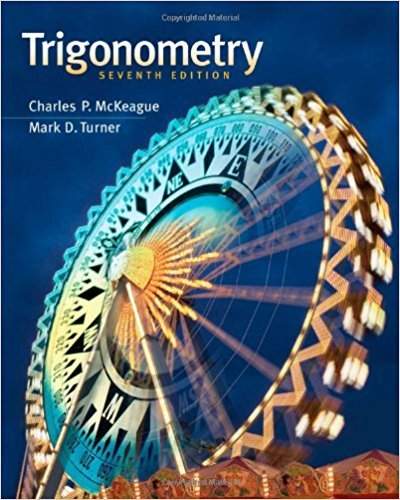×
×

# Solutions for Chapter 6.1: Solving Trigonometric Equations## Full solutions for Trigonometry | 7th Edition

ISBN: 9781111826857Solutions for Chapter 6.1: Solving Trigonometric Equations

Solutions for Chapter 6.1
4 5 0 290 Reviews
14
5
##### ISBN: 9781111826857

This textbook survival guide was created for the textbook: Trigonometry, edition: 7. Chapter 6.1: Solving Trigonometric Equations includes 100 full step-by-step solutions. Trigonometry was written by and is associated to the ISBN: 9781111826857. This expansive textbook survival guide covers the following chapters and their solutions. Since 100 problems in chapter 6.1: Solving Trigonometric Equations have been answered, more than 24696 students have viewed full step-by-step solutions from this chapter.

Key Math Terms and definitions covered in this textbook
• Back substitution.

Upper triangular systems are solved in reverse order Xn to Xl.

• Big formula for n by n determinants.

Det(A) is a sum of n! terms. For each term: Multiply one entry from each row and column of A: rows in order 1, ... , nand column order given by a permutation P. Each of the n! P 's has a + or - sign.

• Circulant matrix C.

Constant diagonals wrap around as in cyclic shift S. Every circulant is Col + CIS + ... + Cn_lSn - l . Cx = convolution c * x. Eigenvectors in F.

A sequence of steps (end of Chapter 9) to solve positive definite Ax = b by minimizing !x T Ax - x Tb over growing Krylov subspaces.

• Fibonacci numbers

0,1,1,2,3,5, ... satisfy Fn = Fn-l + Fn- 2 = (A7 -A~)I()q -A2). Growth rate Al = (1 + .J5) 12 is the largest eigenvalue of the Fibonacci matrix [ } A].

• Fourier matrix F.

Entries Fjk = e21Cijk/n give orthogonal columns FT F = nI. Then y = Fe is the (inverse) Discrete Fourier Transform Y j = L cke21Cijk/n.

• Full column rank r = n.

Independent columns, N(A) = {O}, no free variables.

• Gram-Schmidt orthogonalization A = QR.

Independent columns in A, orthonormal columns in Q. Each column q j of Q is a combination of the first j columns of A (and conversely, so R is upper triangular). Convention: diag(R) > o.

• Hankel matrix H.

Constant along each antidiagonal; hij depends on i + j.

• Indefinite matrix.

A symmetric matrix with eigenvalues of both signs (+ and - ).

• Iterative method.

A sequence of steps intended to approach the desired solution.

• lA-II = l/lAI and IATI = IAI.

The big formula for det(A) has a sum of n! terms, the cofactor formula uses determinants of size n - 1, volume of box = I det( A) I.

• Orthogonal subspaces.

Every v in V is orthogonal to every w in W.

• Random matrix rand(n) or randn(n).

MATLAB creates a matrix with random entries, uniformly distributed on [0 1] for rand and standard normal distribution for randn.

• Saddle point of I(x}, ... ,xn ).

A point where the first derivatives of I are zero and the second derivative matrix (a2 II aXi ax j = Hessian matrix) is indefinite.

• Similar matrices A and B.

Every B = M-I AM has the same eigenvalues as A.

• Special solutions to As = O.

One free variable is Si = 1, other free variables = o.

• Sum V + W of subs paces.

Space of all (v in V) + (w in W). Direct sum: V n W = to}.

• Unitary matrix UH = U T = U-I.

Orthonormal columns (complex analog of Q).